Function Repository Resource:

# PolygonalDiagram

Show an array of polygonal numbers

Contributed by: Wolfram Staff (original work by Eric W. Weisstein)
 ResourceFunction["PolygonalDiagram"][n,m] gives a regular polygon of n sides with m levels of numbers arranged in equally spaced points.

## Details and Options

A polygonal number is an array of n successive natural numbers that can be arranged in a triangle, square, etc. for some values of n, depending on the sides of the polygon.
In general, any n-gonal number is equal to the sum of the (n-1)-gonal number of the same rank and the triangular number of the previous rank.
ResourceFunction["PolygonalDiagram"] accepts the same options as Graphics.

## Examples

### Basic Examples (1)

Get a diagram with a triangle with four levels:

 In:=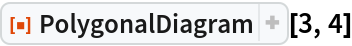Out=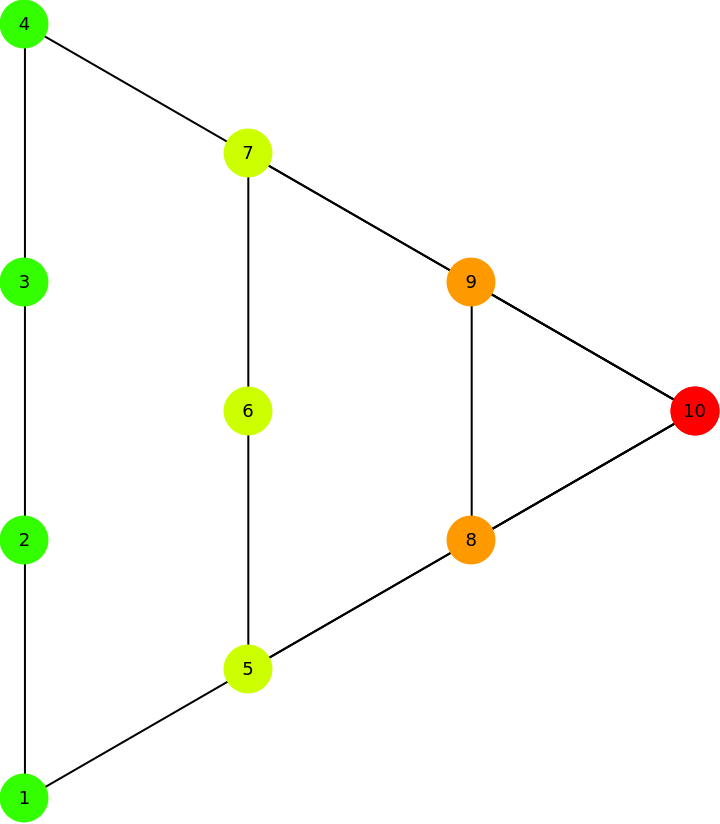### Scope (2)

First five triangular numbers and their diagrams:

 In:=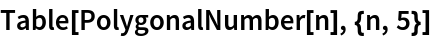Out=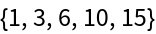In:=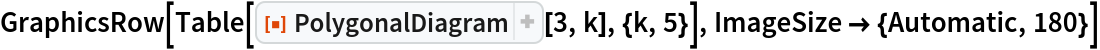Out=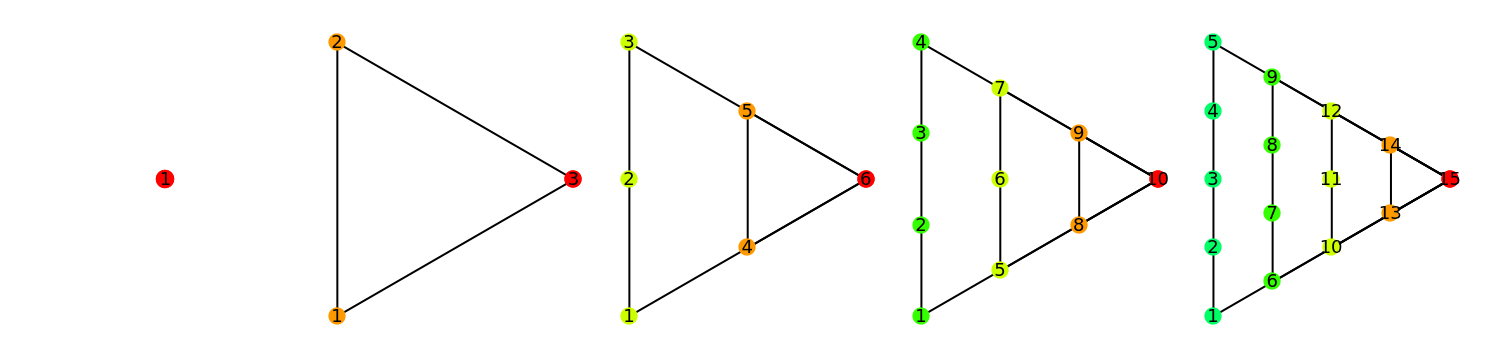Four-level diagrams for various polygonal numbers:

 In:=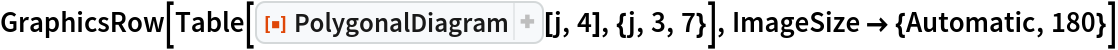Out=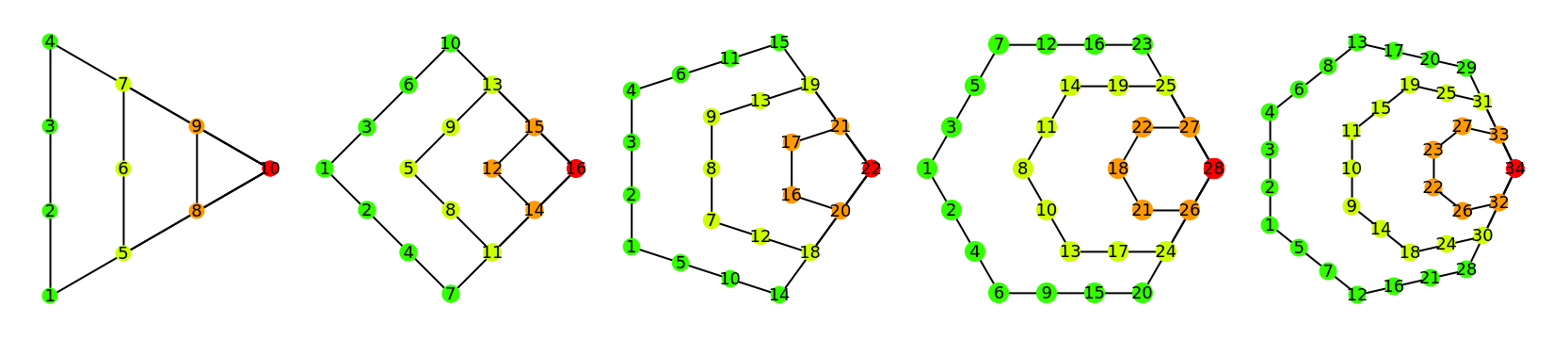### Options (1)

#### PlotLabel (1)

Add a PlotLabel to the diagram:

 In:=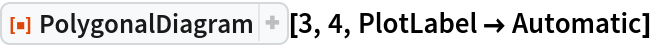Out=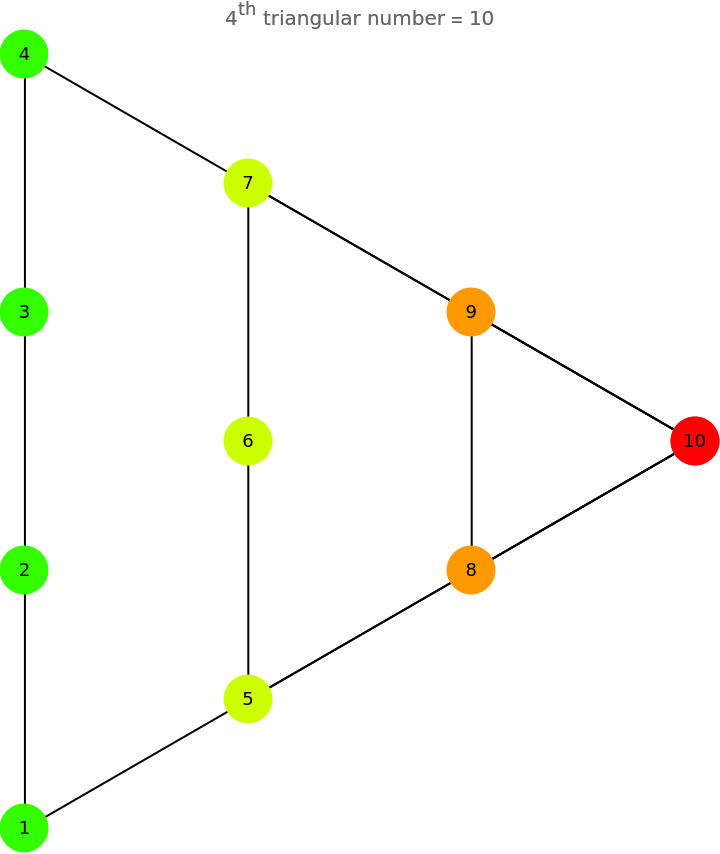### Applications (1)

Demonstrate the addition of polygonal numbers:

 In:=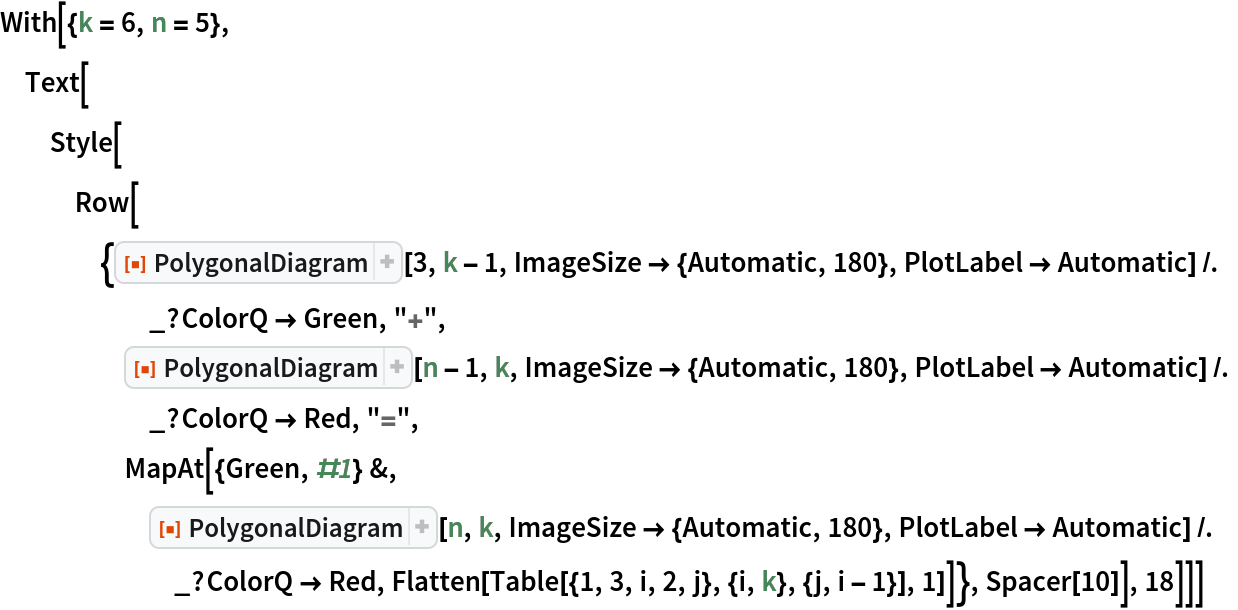Out=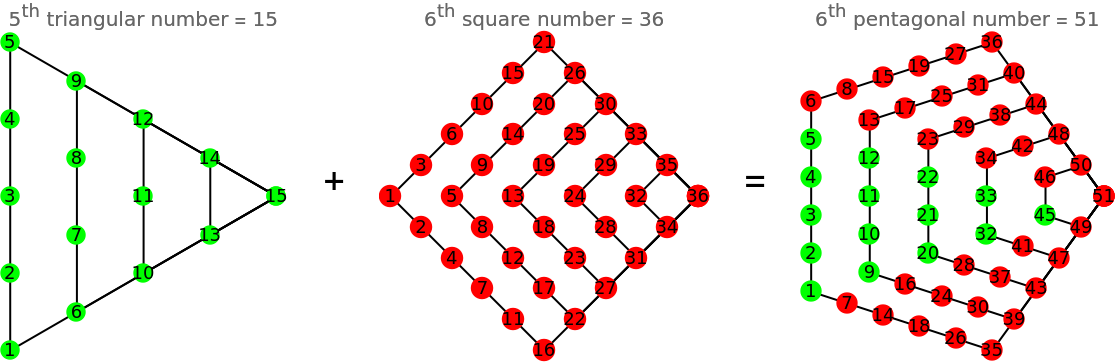## Requirements

Wolfram Language 11.3 (March 2018) or above

## Version History

• 2.0.0 – 14 September 2021
• 1.0.0 – 19 April 2019